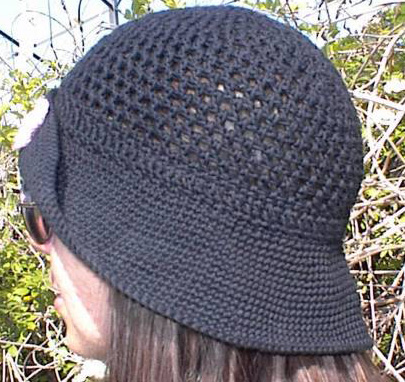# Crocheted Breezy Mesh Hat Pattern## Crocheted Breezy Mesh Hat Pattern

Materials List
Sport-weight Mercerized cotton yarn(SpeedCroSheen)
two 150-yd.balls,hooks "G" & "F"

Guage:With "G" hook,Rnds 1-2 of crown=2 1/4" With "F" hook,5 sc=1"

To Make:
Crown-
Rnd 1:Starting at center,ch 6,s lst to join to form a ring.Ch 4(dc+ch 1),(dc in ring,ch 1)9 times.Join with sl st to third ch of beg ch-4(10 dc).

Rnd 2:Sl st in first ch-1 sp,ch 4,dc in same sp,ch 1,*in next sp work dc,ch 1,dc(V-st made),ch 1.Rep from * 8 more times,join(20 dc).

Rnd 3:Sl st in next ch-1 sp,ch 4,dc in next sp,ch 1,V-st in next sp,ch 1*(dc in next sp,ch 1)twice;V-st in next sp,ch 1.Rep from*to within last 2 sps(dc in next sp,ch 1)twice;join(26 dc).

Rnd 4:Sl st in next sp,ch 4,dc in same sp,ch 1,working in sps,(dc,ch 1)twice,V-st,(dc,ch 1)twice,V-st,(dc,ch 1)3 times,V-st,(dc,ch 1)twice,V-st,(dc,ch 1)3 times,V-st,(dc,ch 1)3 times,V-st,(dc,ch 1)twice,V-st,(dc,ch 1)in last sp,join(34 dc).

Rnd 5:Sl st in next sp,ch 4,work(dc,ch 1)in each sp around,join(34 dc).

Rnd 6:Sl st in next sp,ch 4,dc in same sp,ch 1,(dc,ch 1)4 times,V-st,(dc,ch 1)4 times,V-st,(dc,ch 1)5 times,V-st,(dc,ch 1)4 times,V-st,(dc,ch 1)5 times,V-st,(dc,ch 1)6 times,join(40 dc).

Rnd 7:Sl st in next sp,ch 4,(dc,ch 1)3 times,V-st,*(dc,ch 1)4 times,V-st.Rep from*6 times,join(48 dc).

Rnd 8-15:Sl st in next sp,ch 4,*(dc,ch 1)in next sp.Rep from*around,join.

Note:I thought these symbols might make it easier to understand the stitch placement.Look at each numbered round in a circle.
```I=dc     ^=ch     V=V-st
Rnd 1:I^I^I^I^I^I^I^I^I^I^
Rnd 2:V^V^V^V^V^V^V^V^V^V^
Rnd 3:I^I^V^I^I^V^I^I^V^I^I^V^I^I^V^I^I^V^I^I^
Rnd 4:V^I^I^V^I^I^V^I^I^I^V^I^I^V^I^I^I^V^I^I^I^V^I^I^V^I^
Rnd 5:I^I^I^I^I^I^I^I^I^I^I^I^I^I^I^I^I^I^I^I^I^I^I^I^I^I^I^I^I^I^I^I^I^I^
Rnd 6:V^I^I^I^I^V^I^I^I^I^V^I^I^I^I^I^V^I^I^I^I^V^I^I^I^I^I^V^I^I^I^I^I^I^
Rnd 7:I^I^I^I^V^I^I^I^I^V^I^I^I^I^V^I^I^I^I^V^I^I^I^I^V^I^I^I^I^V^I^I^I^
I^V^I^I^I^I^
Rnd 8-15:I^I^I^I^I^I^I^I^I^I^I^I^I^I^I^I^I^I^I^I^I^I^I^I^I^I^I^I^I^I^I^I^I^I^
I^I^I^I^I^I^I^I^I^I^I^I^I^I^
```

Band-Note:Use a contrasting color for the band,if you like.

Rnd 1:Sl st in next sp,ch 1(sc,ch 1)in each sp around;join(48 sc).

Rnd 2:Rep rnd 1.

Rnd 3:Sl st in next sp,ch 3,dc in same sp,(2 dc in next sp)around;join(96 dc).

Rnd 4:Ch 2,sk next dc,sc in next dc,*Ch 1,sk next dc,sc in next dc.Rep from*around;join(48 sc).

Brim-Note:inc=2 sc in next sc.

Rnd 1:With "F" hook,sc in front loop of next 24 st,inc.Sc in next 48 st,inc.Sc in last 24 st;join(100 sc).

Rnd 2:Ch 1,sc in same st and in next 3 sc,inc.*Sc in next 4 sc,inc.Rep from*around.Sc in last 4 sc;join(120 sc).

Rnd 3:Ch 1,sc in same st and in each sc around;join.

Rnd 4:Ch 1,sc in same st and in next 9 sc,inc.*Sc in next 10 sc,inc.Rep from*around;join(132 sc).

Rnd 5-6:Rep rnd 3.

Rnd 7:Ch 1,sc in same st and in next 10 sc,inc.*Sc in next 11 sc,inc.Rep from*around;join(144 sc).

Rnd 8-9:Rep rnd 3.

Rnd 10:Ch 1,sc in same st and in next 12 sc,inc.*Sc in next 13 sc,inc.Rep from*around.Sc in last 4 sc(154 sc).

Rnd 11-12:Rep rnd 3.

Rnd 13:Ch 1,sc in same st and in next 14 sc,inc.*Sc in next 15 sc,inc.Rep from*around.Sc in last 11 sc;join(164 sc).

Rnd 14-15:Rep rnd 3.

Rnd 16:Ch 1,sc in same st and in next 9 sc,inc.*Sc in next 10 sc,inc.Rep from*around.Sc in last 6 sc;join(174 sc).Fasten off,weave in ends.